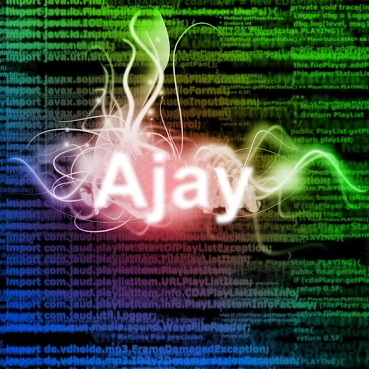Ajay Ramachandran

# Repository

https://github.com/ajayyy/VosterCoasterVR/

# What is Voster Coaster?

Voster Coaster is a VR game where you build roller coasters. I plan on making it a theme park tycoon game in the future as well.

In this update I focused on the ability to form a full roller coaster from parts. To do so, I implemented a system that could take a track piece and bend it to add an incline, or make it a turn piece. This is going to be used to make a full spline-based roller coaster creator in the future.### Bones

3D models can have bones, and adding bones makes it much easier to programatically modify the meshes. In Cinema 4D, I added 10 bones to split each rail into 10 modifiable sections.

### Bones become objects

In Unity, bones get represented as movable objects in the hierarchy. These objects can be moved around just as if they were a normal game object.### Exponential looking curves

In Unity you can set an object's local rotation by using `gameObject.transform.localEulerAngles`. By setting all the objects to have the same local rotation, it creates an exponential looking curve.

The first object is slightly rotated, the next is moved forward in the direction of the previous bone, and then slightly rotated more. This continues for all of the bones

### Targeting an angle

There are 9 usable bones (as the first bone should stay at an angle of 0 to look straight). This means that if you set all of the `gameObject.transform.localEulerAngles` to `targetAngle/9`, it will move toward the target angle.

For example, if the target angle is 90 degrees, you can set each angle to 10 degrees.

``````for (int i = 0; i < rails.Length; i++) {
for (int r = 1; r < rails[i].Length - 1; r++) {
//Attempt to rotate them all
}
}``````

### Length Distortion

By having an exponential, the total distance traveled of the curves will be much smaller than the original. To account for that, a ratio is calculated to figure out how to make the curve reach the full length.

``````//try to stretch the newly shaped incline to the proper size
for (int i = 0; i < rails.Length; i++) {
//get relative total offset for the adjusted track
float difference = rails[i][rails[i].Length - 1].transform.position.z - rails[i].transform.position.z;

float multiplier = sizes[i] / difference;

for (int r = 1; r < rails[i].Length - 1; r++) {
Vector3 pos = rails[i][r].transform.position;

rails[i][r].transform.localPosition *= multiplier;
}
}``````

sizes[i] is a previous value calculated earlier of the original size.

``sizes[i] = rails[i][rails[i].Length - 1].transform.position.z - rails[i].transform.position.z;``

### Turns

For turns, each rail would be a different size, as they have to travel further to reach the same spot (it is like a bigger circle.As you can see in the above image, if the curve is the same size, it will not reach the same point. The orange is where the curve should reach to.

To accommodate for this, the outside circles must be stretched based on their difference from the inside circles.

``````for (int r = 1; r < rails[i].Length - 1; r++) {
if (adjustmentAngle.y != 0) { //making a turn, extend outside curves to accommodate
int insideRail = 0;
insideRail = 1;
}

if (i != insideRail) { //in this case, 1 is the inside rail (doing just zero for now as a test, in the final version it should be based on if the angle is negative or positive)
//get full offset compared to rails[insideRail]
float offset = Mathf.Abs(railParents[insideRail].transform.position.x) + Mathf.Abs(railParents[i].transform.position.x);

}
}
}``````

Inside rail is the index of the inside rail. This will change depending on if the turn is to the left or to the right. This will only happen if the adjustmentAngle is a y value, as y value angles are turns, and x value angles are inclines.

# GitHub Account

https://github.com/ajayyy/

# Pull Request

https://github.com/ajayyy/VosterCoasterVR/pull/3

Small hotfix: https://github.com/ajayyy/VosterCoasterVR/pull/4